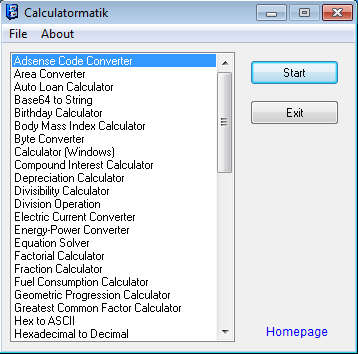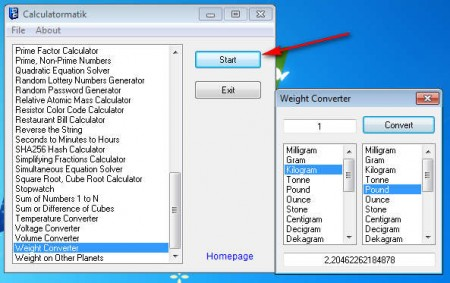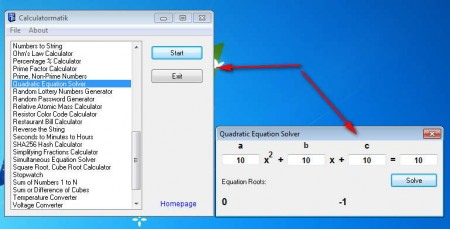Editor Ratings:
User Ratings:
[Total: 0 Average: 0]

Calculatormatik is a free unit converter application which offers a large selection of various different calculators and unit conversion tools. This free unit converter is very small, lightweight and portable, which means that you can run it without installation. This free unit conversion utility offers both metric and royal units, and it can be used to solve simple mathematical equations and calculating your body mass index, among other things.Check out some similar software like: Free Equation Calculator, Magic Plot Free and you may also check out our featured post on 5 Best Free Calculator.

With a size of just 150kb, this free calculator and unit converter will not be too much of a burden for your Windows system. Features which are offered by Calculatormatik are more than enough to satisfy the needs of a large number of different users. This can be seen from the image above, which is the default interface of this free metric unit conversion tool. There’s around 55 different calculations and conversions which can be done with Calculatormatik, here are some of the more interesting ones:

• Area, weight, volume, voltage, current, byte converters, both metric and royal.
• Hex to ASCII and Hexadecimal to Decimal Converters.
• Mortgage, Auto Loan, Fuel Consumption Calculators.
• Simple Equation, Quadratic Equation, Simultaneous Equation Solver.
• Random password and lottery number generators.

These are just some of the functions that you get with Calculatormatik, and as you can see from the list above, conversion isn’t the only thing that this free unit converter can do.

### How to Convert Units with This Free Unit Converter:

Let’s start with unit conversions, more precisely with weight conversion. There’s no particular reason why we chose weight, the conversion works the same for every other unit of measurement. The first step that you need to take is find the weight in the list of possible calculations and conversions.A small drawback of Calculatormatik would be that there’s no grouping of conversion and calculation functions, everything is listed and mixed up together which makes finding the tool you want a bit problematic. When you do find what you need, mark the conversion of calculation with a left mouse click and then click Start. New window should pop-up where you’ll actually do the conversion. See image above.

### How to solve Mathematical Equations with Calculatormatik:

There’s actually a few mathematical equations which are supported, see list above, but for the purpose of this demonstration we’re gonna use quadratic equation.Do the same thing that we did for the weight, but now only select Quadratic Equation Solver from the list. In the window that pops-up enter the numbers for the a,b,c and the solution, hit solve. Program is gonna present you with the solutions of the equation, if it has a solution.

### Conclusion

These simple conversions and calculations that we showed here are just the tip of the iceberg. A lot more can be done with Calculatormatik and if you are looking for a way of how you can combine all of your unit conversions, both royal and metric, and of course calculation also, then this free utility is just the thing for you. If you’re skeptical, then just try it out, it’s portable and very small, you won’t have to wait long for download and not at all when it comes to installation.